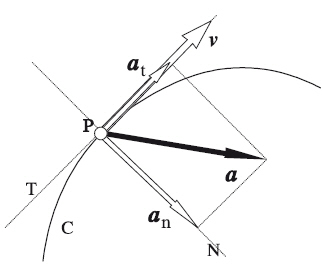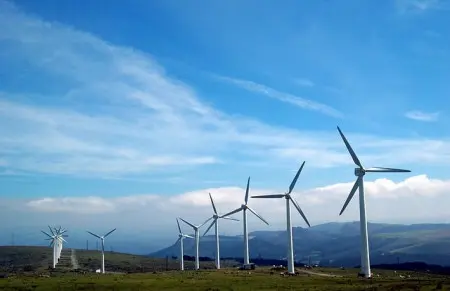# Uniformly Accelerated Circular Motion - MCUAUniformly accelerated circular motion (UACM) is a type of motion in which an object moves along a circular path with a constant acceleration.

The MCUA is a type of movement crucial in calculating the kinematics of a body. This phenomenon is fundamental to understanding a wide range of situations, from vehicle mechanics to the dynamics of orbiting planets, and can be described by specific formulas that relate angular velocity, angular acceleration, and time.

## What Is Uniformly Accelerated Circular Motion?

Uniformly accelerated circular motion (UACM) is a fundamental concept in physics that describes the motion of an object along a circular path with a constant acceleration.

Unlike rectilinear motion, in which an object moves in a straight line, MCUA involves a constant variation in the angular velocity of the object, causing it to rotate along a closed curve.

In the MCUA, the angular acceleration of the object is constant, meaning that its angular velocity increases or decreases uniformly with time.

## Basic Characteristics of the MovementThe basic characteristics that define this movement are:

• The object moves in a circular path. This implies the appearance of a centripetal acceleration to keep the distance of the object from the center of rotation constant.
• Angular velocity undergoes a constant angular acceleration, therefore, tangential velocity also undergoes a tangential acceleration.

## Basic Concepts

### Angular Velocity

Angular velocity, represented by the Greek letter "ω" (omega), is a measure of how quickly an object rotates along the circular path. It is measured in radians per second (rad/s).

### Tangential Speed

Tangential velocity is the linear velocity of an object at a specific point on its circular path. It refers to the magnitude of the velocity of an object in a direction tangent to the curve at that point.

### Angular Acceleration

Angular acceleration, represented by "α" (alpha), is the change in angular velocity per unit time. Like angular velocity, it is measured in radians per second squared (rad/s²).

### Centripetal Acceleration

Centripetal acceleration is an acceleration directed toward the center of a circular path. It occurs when an object moves in a curved orbit and experiences a constant change in the direction of its speed without affecting its magnitude.

### Tangential Acceleration

Tangential acceleration is a measure of how the magnitude of the speed of a moving object changes along a curved path. This acceleration occurs when the tangential speed of the object varies.

## Calculation Formulas

### Formula for Angular Velocity as a Function of Time

The relationship between initial angular velocity (ω₀), angular acceleration (α) and time (t) can be described by the following formula:

ω= ω₀ + α·t

Where

• ω is the final angular velocity.
• ω₀ is the initial angular velocity.
• α is the angular acceleration.
• t is time

### Rotated Angle Formula

The amount of angle rotated (θ) at a time "t" is related to the angular velocity and angular acceleration as follows:

θ = ω₀·t + ½·α·t²

Where

• θ is the rotated angle.
• ω₀ is the initial angular velocity.
• α is the angular acceleration.
• t is time

### Formula for Angular Velocity as a Function of Angular Velocity

The final angular velocity (ω) is related to the initial angular velocity, the angular acceleration and the rotated angle by the following formula:

ω² = ω₀² + 2·α·θ

Where

• ω is the final angular velocity.
• θ is the rotated angle.
• ω₀ is the initial angular velocity.
• α is the angular acceleration

## Illustrative Everyday ExamplesUniformly accelerated circular motion (UACM) is found in numerous aspects of everyday life and in various contexts, some examples include:

• Clothes Washer: In the spin cycle, clothes spin in the drum with a constant acceleration, allowing water to be expelled out.
• Fairground rides: Fairground rides, such as Ferris wheels and carousels, apply a start-stop MCUA to allow passengers to get on and off.
• Wind turbines: windmills suffer a MCUA when they start up or when there are variations in wind intensity.
• Steam turbines: The turbines of a nuclear power plant are optimized to rotate at a constant speed. While uniformly accelerated circular motion occurs during starting and stopping, its efficiency is lower.

## Solved Exercises

### Exercise 1: Rotation of a FanA fan rotates with an initial angular velocity of 3 rad/s and experiences an angular acceleration of 0.5 rad/s². How long will it take to reach an angular velocity of 7 rad/s?

Solution

We use the formula for the final angular velocity:

ω = ω₀ + αt

We substitute the known values:

We solve for you:

The fan will take 8 s to reach an angular velocity of 7 rad/s.

### Exercise 2: Spin of a Ferris Wheel

A ferris wheel begins to rotate from rest with an angular acceleration of 0.8 rad/s². How long will it take for the ferris wheel to turn 5 radians?

Solution

We use the formula for the rotated angle:

θ = ω₀t + ½·α·t²

We substitute the known values:

We simplify the equation: